## Example Questions

### Example Question #1 : How To Divide Integers

A car averages 29 miles per gallon.  If gas costs $3.75 per gallon how much money would need to be spent on gas to travel 1464.5 miles? Possible Answers:$189.38

$5491.88$50.5

$108.75 Correct answer:$189.38

Explanation:

The question is asking for the amount of money that would need to be spent on gas.  Using the value given for the miles per gallon of the car and the amount of miles traveled it is possible to determine the gallons of fuel that will be required.  This will be set up as 1464.5 miles travelled divided by the 29 miles per gallon that the car gets.  This leaves us with 50.5 gallons of fuel used.  From this point we can multiply the amount of fuel used, 50.5 gallons, by the price of fuel per gallon, $3.75, to obtain the amount of money that will spent on fuel,$189.38.

### Example Question #1 : Operations

Connie's car gets 35 miles per gallon of gas.  How much gas will she need to take a 525 mile trip?  Round to the nearest gallon.

15

20

23

17

25

15

Explanation:

This becomes a division problem: 525 miles ÷ 35 miles per gallon = 15 gallons

### Example Question #3 : How To Divide Integers

A father buys a bag of marbles.  He divides the marbles among his 5 children, who receive 24 marbles each. If there had been 6 children, how many marbles would each one get?

22

24

20

18

20

Explanation:

There were a total of 120 marbles to begin with, since 5 * 24 = 120.

If the marbles are split between 6 children, then each child gets 120/6 = 20 marbles each.

### Example Question #4 : How To Divide Integers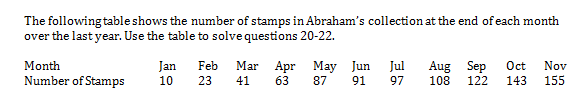At the end of December, Abraham adds the average number of stamps he has been collecting over the year to his album, how many stamps will he have at the end of December?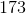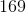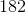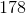In 11 months, Abraham has collected 155 stamps, or an average of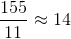stamps per month. At the end of December, he should have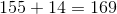stamps.